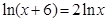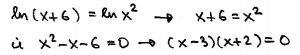In a discussion during the conference, the term “math maturity” was mentioned.  We agreed that it is a poorly defined term and could mean lots of different things.

In my view, math maturity refers to the growth of an individual as a mathematician, and is based on their experiences, insights and reflection. Developed by repeated exposure to mathematics, math maturity includes (but is not limited to) ability to read and understand math notation and work with math symbols; capacity to handle abstract ideas, and to generate abstractions; ability to ask right questions; ability to reason logically and to produce a coherent mathematical narrative.

Instead of going into details here, I will illustrate what I mean in a couple of examples. In my experience (and that of my colleagues), even our better math students, after taking a number of math courses, do not possess those extra qualities that would qualify them as math mature.

Elementary example. When we ask students to solve the equationwe see the usual sequence of stepsfrom which many conclude that there are two solutions, x=3 and x=-2. Very few students note that x cannot be negative. (Math maturity: no matter what equation you are solving, check whether or not solutions make sense.)

Advanced example, coming from my third-year problem solving course. A typical student in this course has taken a minimum of 6-7 university math courses. To start, I ask my students to write a code (in Python) to generate a list of first one million prime numbers. Pointing that this is really cool and exciting (as only 20 years ago producing such a list would take lot more effort and time), students work on a problem sheet, which suggests ideas they can investigate. The last question asks them to identify interesting facts about prime numbers, and/or the prime number counting function, that they discovered on their own. Here is a sample of their complete answers:

“The numbers increase exponentially”

“There are less and less prime numbers”

“All prime numbers are relatively prime”

“The only even prime number is 2; no prime greater than 5 ends with 5. I googled these”

I do not think that anyone would call these math-mature answers.